# Samacheer Kalvi 9th Maths Book Solutions Chapter 8 | Statistics Answers For Tamil Nadu Board

Samacheer Kalvi 9th Maths Book Solutions Chapter 8 – Statistics is available here. The Samacheer Kalvi 9th Maths book answers of Chapter 8, available at BYJU’S, contain step by step explanations designed by our Mathematics experts. All these important questions are based on the new pattern prescribed by the Tamil Nadu board. Students can also get the solutions of other chapters on Samacheer Kalvi 9th Maths solutions. Students can solve these Samacheer Kalvi Class 9 Maths Book Chapter 8 Questions for practise and then refer to its solutions to get an idea about their exam preparations.

## Samacheer Kalvi Class 9 Maths Book Chapter 8 Questions with Solutions

Chapter 8 of the Samacheer Kalvi 9th Maths guide will help the students to solve problems related to the collection of data, measures of central tendency, arithmetic mean, the median, the mode, relationship between mean, median and mode.

### Samacheer Kalvi 9th Maths Chapter 8: Statistics Book Exercise 8.1 Questions and Solutions

Question 1: In a week, the temperature of a certain place is measured during winter as follows 26oC, 24oC, 28oC, 31oC, 30oC, 26oC, 24oC. Find the mean temperature of the week.

Solution:

Mean temperature

= Sum of all temperature / Number of terms

= (26 + 24 + 28 + 31 + 30 + 26 + 24) / 7

= 189 / 7

= 27

The mean temperature is 27oC.

Question 2: The mean weight of 4 members of a family is 60 kg. Three of them have a weight of 56 kg, 68 kg and 72 kg, respectively. Find the weight of the fourth member.

Solution:

Let x be the weight of the fourth member.

Mean weight of 4 members = 60 kg

(56 + 68 + 72 + x) / 4 = 60

196 + x = 60(4)

x = 240 – 196

x = 44 kg

The weight of the fourth member is 44 kg.

Question 3: In a class test in mathematics, 10 students scored 75 marks, 12 students scored 60 marks, 8 students scored 40 marks and 3 students scored 30 marks. Find the mean of their score.

Solution:

Number of students scored 75 marks = 10

Number of students scored 60 marks = 12

Number of students scored 40 marks = 8

Number of students scored 30 marks = 3

= [10(75) + 12(60) + 8(40) + 3(30)] / (10 + 12 + 8 + 3)

= (750 + 720 + 320 + 90) / 33

= 1880 / 33

= 56.96

Question 4: In a research laboratory scientists treated 6 mice with lung cancer using natural medicine. Ten days later, they measured the volume of the tumour in each mouse and given the results in the table. Find the mean.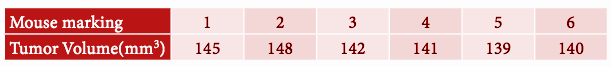Solution:

= (145 + 148 + 142 + 141 + 139 + 140) / 6

= 855 / 6

= 142.5 mm3

Question 5: If the mean of the following data is 20.2, then find the value of p.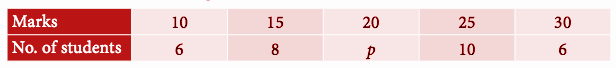Solution:

 x f fx 10 6 60 15 8 120 20 p 20p 25 10 250 30 6 180 Total 30 + p 610 + 20p

Mean = (610 + 20p) / (30 + p)

Mean = 20.2

(610 + 20p) / (30+p) = 20.2

610 + 20p = 20.2 (30+p)

610 + 20 p = 606 + 20.2p

20.2p – 20p = 610 – 606

0.2p = 4

p = 4 / 0.2

p = 20

Question 6: In the class, the weight of students are measured for the class records. Calculate the mean weight of the class students using the direct method.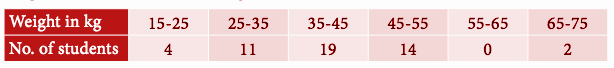Solution:

 Weight Number of students Midpoint fx 15 – 25 4 20 80 25 – 35 11 30 330 35 – 45 19 40 760 45 – 55 14 50 700 55 – 65 0 60 0 65 – 75 2 70 140 Total 50 2010

Mean = 2010 / 6 = 335

Question 7: Calculate the mean of the following distribution using Assumed Mean Method.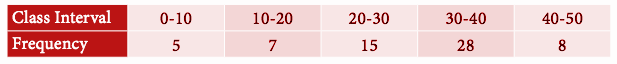Solution:

A = 25

 Class Interval Frequency Midpoint d = x – 25 fd 0 – 10 5 5 -20 -100 10 – 20 7 15 -10 -70 20 – 30 15 25 0 0 30 – 40 28 35 10 280 40 – 50 8 45 20 160 63 270

Mean = A + ∑ fd / ∑ f

= 25 + (270 / 63)

= 25 + 4.28

Mean = 29.28

Question 8: Find the Arithmetic Mean of the following data using Step Deviation Method: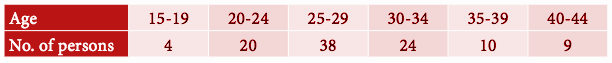Solution:

 Age Frequency Midpoint d = x – 25 fd 14.5 – 19.5 4 17 -3 -12 19.5 – 24.5 20 22 -2 -40 24.5 – 29.5 38 27 -1 -38 29.5 – 34.5 24 32 0 0 34.5 – 39.5 10 37 1 10 39.5 – 44.5 9 42 2 18 105 -62

Mean = A + (∑ fd / ∑f) x c

= 32 + (-62 / 105) x 5

= 32 – 0.59 (5)

= 32 – 2.95

Mean = 29.05

### Samacheer Kalvi 9th Maths Chapter 8: Statistics Book Exercise 8.2 Questions and Solutions

Question 1: Find the median of the given values : 47, 53, 62, 71, 83, 21, 43, 47, 41.

Solution:

Arranging the given data in ascending order

21, 41, 43, 47, 47, 53, 62, 71, 83

Number of values given = 9 (odd)

Median = [(N + 1) / 2]th value

Median = 10 / 2 = 5th value.

Hence the median is 47.

Question 2: Find the median of the given data: 36, 44, 86, 31, 37, 44, 86, 35, 60, 51

Solution:

Arranging the given data in ascending order,

31, 35, 36, 37, 44, 44, 51, 60, 86, 86

Number of given observations = 10

Median = (10 / 2)th value + [(10 / 2) + 1]th value

= (5th value + 6th value) / 2

= (44 + 44) / 2

= 44

Question 3: The median of observation 11, 12, 14, 18, x + 2, x + 4, 30, 32, 35, 41 arranged in ascending order is 24. Find the values of x.

Solution:

The data given is in ascending order.

The number of observations of the given data is 10

Median is average of 5th and 6th observations.

[(x + 2) + (x + 4)] / 2 = 24

2x + 6 = 48

2x = 48 – 6

2x = 42

x = 21

Question 4: A researcher studying the behaviour of mice has recorded the time (in seconds) taken by each mouse to locate its food by considering 13 different mice as 31, 33, 63, 33, 28, 29, 33, 27, 27, 34, 35, 28, 32. Find the median time that mice spent in searching for its food.

Solution:

31, 33, 63, 33, 28, 29, 33, 27, 27, 34, 35, 28, 32

The ascending order of given data is

27, 27, 28, 28, 29, 31, 32, 33, 33, 33, 34, 35, 63

The middle value is 7th observation

Median = 32

Question 5: The following are the marks scored by the students in the summative assessment exam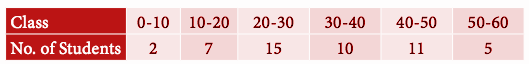Find the median.

Solution:

 Class Number of students Cumulative Frequency 0 – 10 2 2 10 – 20 7 9 20 – 30 15 24 30 – 40 10 34 40 – 50 11 45 50 – 60 5 50 = N

N / 2 = 50 / 2 = 25th value

Median class = 20 – 30

l = 20, N / 2 = 25, m = 9, f = 15 and c = 10

Median = 20 + ([25 – 9] / 15) x 10

= 20 + (16 / 15) x 10

= 20 + 1.06 (10)

= 20 + 10.6

= 30.6

Hence, the median of the given data is 31.

Question 6: The mean of five positive integers is twice their median. If four of the integers are 3, 4, 6, 9 and median is 6, then find the fifth integer.

Solution:

Let x be the fifth observation.

Mean of 5 observations = (3 + 4 + 6 + 9 + x) / 5

= (22 + x) / 5

Median = 6

Mean = 2 median

(22 + x) / 5 = 2 (6)

22 + x = 12 (5)

22 + x = 60

x = 60 – 22

x = 38

Hence, the 5th observation is 38.

### Samacheer Kalvi 9th Maths Chapter 8: Statistics Book Exercise 8.3 Questions and Solutions

Question 1: The monthly salary of 10 employees in a factory are given below :

₹ 5000, ₹ 7000, ₹ 5000, ₹ 7000, ₹ 8000, ₹ 7000, ₹ 7000, ₹ 8000, ₹ 7000, ₹ 5000.

Find the mean, median and mode

Solution:

Mean = (5000 + 7000 + 5000 + 7000 + 8000 + 7000 + 7000 + 8000 + 7000 + 5000) / 10

= 66000 / 10

= 6600

Median:

5000, 5000, 5000, 7000, 7000, 7000, 7000, 7000, 8000, 8000

Number of observations = 10 (Even)

Median = {(10 / 2)th observation + [(10 / 2) + 1]th} / 2

= (5th observation + 6th observation) / 2

= (7000 + 7000) / 2

= 14000 / 2

= 7000

Mode: 7000 is repeating 5 times. Hence, mode is 7000.

Question 2: Find the mode of the given data: 3.1, 3.2, 3.3, 2.1, 1.3, 3.3, 3.1

Solution:

3.1 and 3.3 are repeating twice, so the mode is 3.1 and 3.3

It is bimodal data.

Question 3: For the data 11, 15, 17, x + 1, 19, x – 2, 3 if the mean is 14 , find the value of x. Also, find the mode of the data.

Solution:

Mean = (11 + 15 + 17 + x + 1 + 19 + x – 2 + 3) / 7

14 = (64 + 2x) / 7

14(7) = 64 + 2x

2x = 98 – 64

2x = 34

x = 34 / 2 = 17

By applying the value of x in the given observation,

11, 15, 17, 18, 19, 15, 3

Mode = 15 (Repeating twice)

Question 4: The demand for tracksuit of different sizes as obtained by a survey is given below: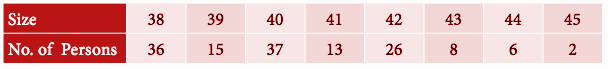Which size is in greater demand?

Solution:

The demand for size 40 is 37.

So, the demand for size 40 is high.

Question 5: Find the mode of the following data: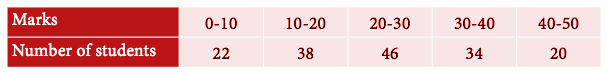Solution:

The highest frequency is 46.

Modal class is 20 – 30.

l = 20, f = 46, f1 = 38, f2 = 34, c = 10

= 20 + [(46-38)/2(46) – 38 – 34] x 10

= 20 + [8/(92 – 38 – 34)] x 10

= 20 + [8/20] x 10

= 20 + 4

= 24

Hence, the mode is 24.

Question 6: Find the mode of the following distribution: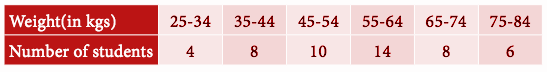Solution:

 Weight (in kgs) Number of students 24.5 – 34.5 4 34.5 – 44.5 8 44.5 – 54.5 10 54.5 – 64.5 14 64.5 – 74.5 8 74.5 – 84.5 6

The highest frequency is 14

modal class is 54.5 – 64.5

l = 54.5, f = 14, f1 = 10, f2 = 8, c = 10

= 54.5 + [(14 – 10) / 2 (14) – 10 – 8] x 10

= 54.5 + [4 / (28 – 18)] x 10

= 54.5 + [4 / 10] x 10

= 54.5 + 4

= 58.5

Hence, the mode is 58.5.